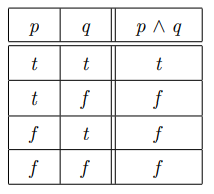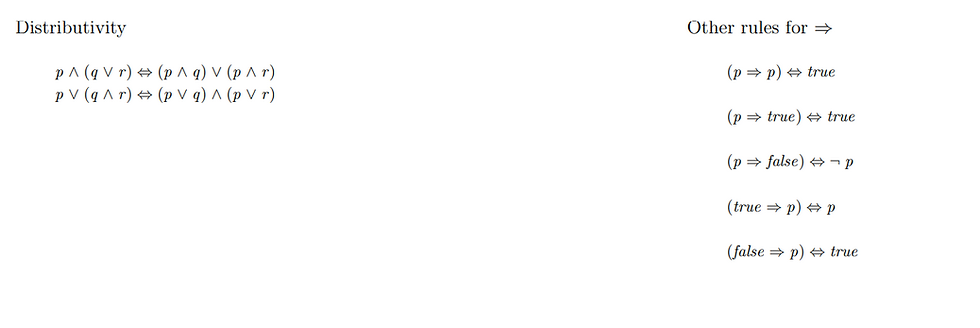top of page
Search

# Discrete Mathematics Homework Help | Software Engineering Mathematics

### Introduction And Propositions

In this tutorial, we will study the heart of discrete mathematics:

• propositional logic: making statements

• set theory: describing collections of objects

• predicate logic: making statements about objects

• relations, functions, sequences: describing relationships between objects

• recursion and induction: reasoning about repeated application (and returning definitions

Contents

• Discrete mathematics

• The Z notation

• Propositions

• Tautologies

• Equivalences

The Z notation

The syntax and semantics that we choose for discrete mathematics is that of the Z notation:

• The logic is typed: every identifier in our mathematical document is associated with a unique basic set

• Functions are partial by default: the result of applying a function to a particular object may be undefined

• The various sub-languages are precisely defined: a Z document is easily parsed and type-checked

Propositions

A proposition is a statement that must be either true or false Note that we deal with a two-valued logic (cf. SQL) Propositions may be combined using logical connectives The meaning of a combination is determined by the meanings of the propositions involved.

Examples

• 2 is even

• 2 + 2 = 5

• tomorrow = tuesday

• she is rich

• he is tall

• 2 / 0 = 0

Examples

• ¬ (2 is an even number)

• she is rich ∧ he is tall

• the map is wrong ∨ you are a poor navigator

• (2 + 2 = 5) ⇒ (unemployment < 2 million)

• (tomorrow = tuesday) ⇔ (today = monday)

Truth Table

We use truth tables to give a precise meaning to our logical connectivePractice Example: